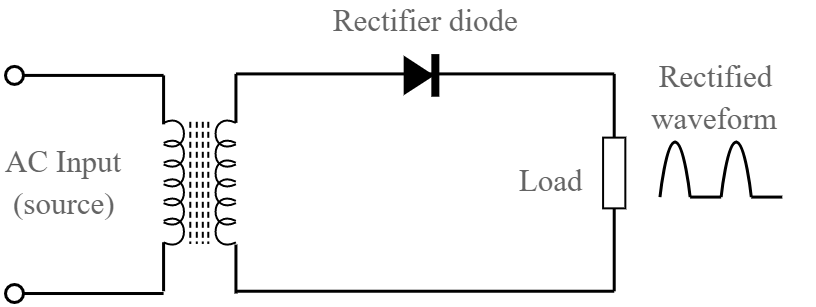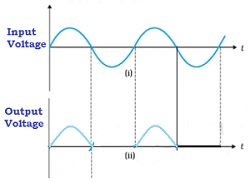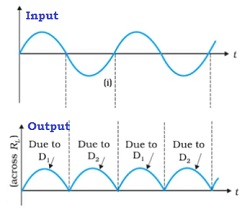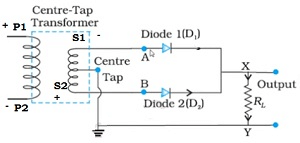NEET  >  Diode as a Rectifier

# Diode as a Rectifier Notes | Study Physics Class 12 - NEET

## Document Description: Diode as a Rectifier for NEET 2022 is part of Physics Class 12 preparation. The notes and questions for Diode as a Rectifier have been prepared according to the NEET exam syllabus. Information about Diode as a Rectifier covers topics like Application of junction diode as a Rectifier, Half-wave Rectifier, Full Wave Rectifier and Diode as a Rectifier Example, for NEET 2022 Exam. Find important definitions, questions, notes, meanings, examples, exercises and tests below for Diode as a Rectifier.

Introduction of Diode as a Rectifier in English is available as part of our Physics Class 12 for NEET & Diode as a Rectifier in Hindi for Physics Class 12 course. Download more important topics related with notes, lectures and mock test series for NEET Exam by signing up for free. NEET: Diode as a Rectifier Notes | Study Physics Class 12 - NEET
 Table of contents Application of junction diode as a Rectifier Half-wave Rectifier Full Wave Rectifier
 1 Crore+ students have signed up on EduRev. Have you?

Application of junction diode as a Rectifier

• Rectifier is a device which is used for converting alternating current or voltage into direct current or voltage
• A p-n junction diode can be used as a half-wave and full-wave rectifier
• The resistance of a p-n junction diode becomes low when forward biased and becomes high when reverse biased. This is the principle of the working of rectifier

Half-wave Rectifier

Circuit Diagram• Transformer with primary and secondary coils
• Diode
• Load resistance RL

The AC voltage to be rectified is connected between the primary of the transformer
To one coil of the secondary, the p junction of the diode is connected
The output is measured across the load resistance RL
Input and outputWorking

Case 1

• During the positive half cycle of the input AC voltage, suppose P1 is negative and P2 is positive
• On account of inductance, S1 becomes positive and S2 becomes negative
• The p-n junction is forward biased and hence the resistance of the p-n junction diode becomes low
• Hence, current flows in the circuit and we get output across the load resistance RL
• This is indicated in the graph above

Case 2

• During the negative half cycle of the input AC voltage, suppose P1 is positive and P2 is negative
• On account of inductance, S1 becomes negative and S2 becomes positive
• The p-n junction is reverse biased and hence the resistance of the p-n junction diode becomes high
• Hence, no current flows in the circuit and we do not get any output across the load resistance RL
• This is indicated in the graph above

The above process is repeated. Thus , we have current only in the positive half of the cycle. Hence, if is called as half-wave rectifier
The output signal is not continuous and available as bursts. Hence, this is not of much use.

Full Wave Rectifier

Circuit Diagram• The main difference between half and full wave rectifier in circuit, is the usage of two diodes – D1 and D2

Input and OutputWorking

Case 1

• During the positive half of the input cycle of AC voltage, the junction diode D1 is forward biased as shown in the diagram above
• Hence, current flows in the above circuit as indicated
• The diode D2 is reverse biased and hence no current due to D2
• We get output when the same is measured across the load resistance RL due to the diode D1 alone

Case 2

• The circuit diagram for the negative half of the input cycle of AC voltage:• During the positive half of the input cycle of AC voltage, the junction diode D2 is forward biased as shown in the diagram above.
• Hence, current flows in the above circuit as indicated.
• The diode D1 is reverse biased and hence no current due to D1.
• We get output when the same is measured across the load resistance RL due to the diode D2 alone

We observe that one of the diode conducts and the flow of current across the load resistance is in the same direction Also, current flows during both cycles of the input AC voltage. However, the output though unidirectional has ripple contents. Ripple contents indicate both AC and DC components
We can get only the DC component by passing it through a filter circuit
The filter circuit consists of Resistance and Capacitance

Circuit Diagram

• C has a high capacitance value and serves as a filter circuit
• RL is a load resistance

Working

• The capacitance offers low impedance to AC component and offers infinite impedance to DC component
• Due to this the AC component is bypassed or filtered out
• The DC component produces a voltage drop across the load resistance which is almost DC voltage

Problem: In half-wave rectification, what is the output frequency if the input frequency is 50 Hz. What is the output frequency of full wave rectifier for the same input frequency?
Solution: Half-wave rectifier –The output voltage is obtained is once in one cycle of input voltage, hence output ripple frequency after half-wave rectification = 50 Hz
Full-wave rectifier – For one cycle of input voltage, we get output twice in the same direction. Hence, the output after full wave rectification = 100 Hz

The document Diode as a Rectifier Notes | Study Physics Class 12 - NEET is a part of the NEET Course Physics Class 12.
All you need of NEET at this link: NEET

## Physics Class 12

157 videos|425 docs|213 tests
 Use Code STAYHOME200 and get INR 200 additional OFF

## Physics Class 12

157 videos|425 docs|213 tests

### How to Prepare for NEET

Read our guide to prepare for NEET which is created by Toppers & the best Teachers

Track your progress, build streaks, highlight & save important lessons and more!

,

,

,

,

,

,

,

,

,

,

,

,

,

,

,

,

,

,

,

,

,

;